# Symmetric Encryption Cryptography in Java

• Last Updated : 25 Aug, 2021

Cryptography is the study of different techniques to secure data from an unauthorized entity. In computer science, we try to develop strategies and practices for protecting sensitive data. Most of the cryptography involves very advanced Mathematical functions used for securing data. The sole purpose of the algorithms developed for cryptography is to hide data from the attacker or middleman. In this article, we will understand the concept in cryptography named symmetric encryption.
Before getting into the concept, lets first understand a few key terms involved in cryptography. They are:

• Plain text: The plain text is the original message or data that is hidden from view through a cryptographic encryption algorithm.
• Cipher text: It is the output of Encryption operation when given key and plain text. It is also the data fed to a Decryption function.
• Key: The key is a piece of data or correlated pair of data when input with plain text into an encryption function yields ciphertext. The key must be secured as the algorithm is publicly available.

• Salt: A salt is a random piece of data when added to a hashing function, provides even more entropy in the output, making attacks to happen less likely. A good cryptographic hash should always use salt.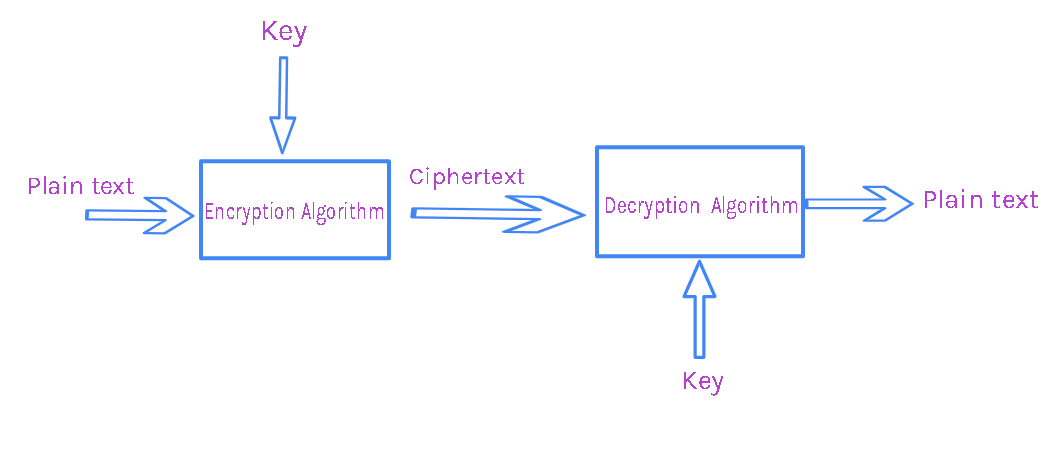Now, lets understand the functions of the components involved in cryptography.

• Digital signature: A digital signature is a function that verifies the authenticity of a message coming remotely. By signing the message with the private key, using the public key to decrypt the message confirms the sender’s authenticity.
• Encryption: Encryption is the process of taking plain text and converting it to a ciphertext, which again can be converted back to original data with an appropriate key at a later point. It is either accomplished with a shared key or an asymmetric key pair.
• Hashing: hashing allows us to map an arbitrary sized set of bytes into a finite size of a relatively unique set of bytes. It is a one-way mathematical function to map data.

Java Cryptography Architecture
The JCA(Java Cryptography Architecture) is the heart and soul of the java encryption, decryption, hashing, secure random, and several other engines that allow us to do cryptographic functions with java programming. The following are a few basic concepts involved in this architecture.

• Secure Random: This engine is used to create cryptographically strong random numbers. Secure Random is an essential requirement for good crypto operations in java. It is either used for seeds or keys.
• Message Digest: This engine is used to produce cryptographically secure hashes. The digest is a hash function that takes variable-length input and produces fixed-length output.
• Signature: It is an engine to create and validate digital signatures. Digital signatures are unique they take a combination of a hash and a public key encryption asymmetric operation to create a unique value or signature.
• Cipher: It is the engine to provide encryption operations. A cipher provides both support for asymmetric and symmetric operations. A cipher also supports stream and block ciphers.

Encryption: Encryption is the process of hiding plaintext data with the use of a key, which yields ciphertext. The Ciphered text becomes difficult to read without the key that was used to generate that ciphertext. Only the authorized entities can read the original text. Lets understand the goals of the encryption. In the coming illustrations, we are considering “A” as the sender and “B” as the receiver.

• Confidentiality: It is also known as privacy that is the message that “A” sends to “B” is only readable by “B” assuming that the algorithm and key is shared by only “A” and “B.”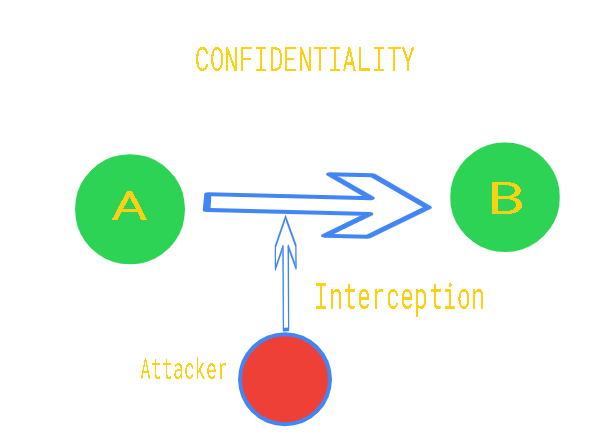• Integrity: The data sent by “A” should receive to “B” without any modification by a bad actor or any middleman attacks.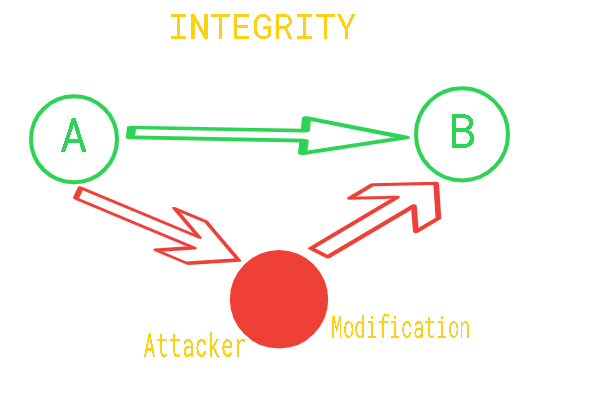• Authentication: It is the process of verifying or recognizing the user’s identity using cryptography. For example, if “A” sends a message to “B”, then authenticity is proving that the message was sent by “A” only.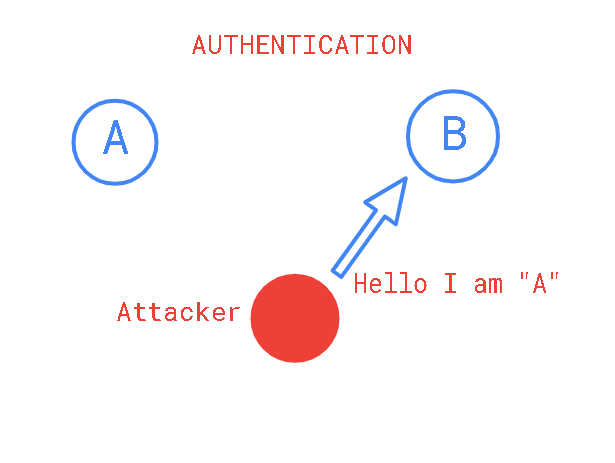• Nonrepudation: It is a way of proving that message came from “A” and “B” can believe that it came from “A” and “A” can confirm that he sent it.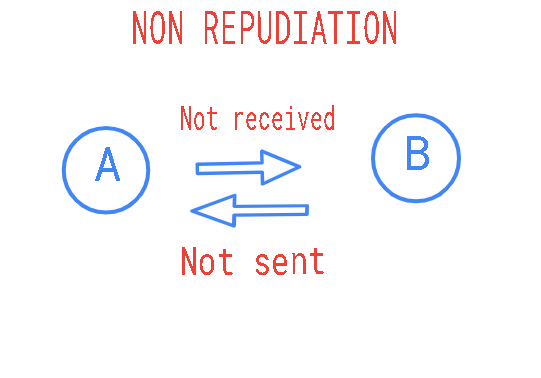Symmetric Encryption: Symmetric encryption or shared key encryption is a method of encryption where both the parties involved share a standard key. That common key must be kept secret by both the parties. For example, “A” will encrypt a message with a shared key “K, ” then “B” can decrypt the encrypted message only with “K.”
Asymmetric Encryption: Asymmetric encryption or public/private key pair encryption is based on the concept of two keys that are mathematically related, (i.e.), one to encrypt and the other to decrypt. For example, “A” will create a public and private key, and he will share the public key with everyone. He can encrypt a message with the private key and send it to “B.” “B” can decrypt the message using “A’s” public key, which is openly available. If “B” wants to send a private message to “A, ” he can encrypt the data using “A’s” public key, and “B” can then decrypt it using his private key.
Implementing Symmetric Encryption
In order to implement symmetric encryption, we need a shared key. We will generate a shared key, but before that, we need to know a few classes and functions. They are:

1. Class SecureRandom: This class helps generate a secure random number. The following are the constructor details of the class:
• SecureRandom() constructs a random secured number generator using default random number algorithm.
• public SecureRandom(byte[] seed) SecureRandom instance is seeded with the specified seed bytes. The parameters required by this constructor is a seed.
• protected SecureRandom(SecureRandomSpi secureRandomSpi, Provider provider). The parameters of this constructor is secureRandomSpi which refers to the SecureRandom implementation. and provider which represents the provider.
• getInstance(): Returns a SecureRandom object and applies random number generation algorithm. The syntax of this method is:
<blockquote
public static SecureRandom getInstance(String algo) throws NoSuchAlgorithmException

• getProvider(): This method returns a SecureRandom object. The syntax of this method is:

public final Provider getProvider()

• getAlgorithm(): This method returns the algorithm name implemented by the SecureRandom object. The syntax of this method is:

public String getAlgorithm()

• setSeed(): This method reseeds the random object. The given seed is added, rather than replacing the existing seed. Thus helping randomness. The syntax of this method is:

public void setSeed(byte[] seed)

• getSeed(): This method returns the given number of seed bytes, calculated by using the seed generation algorithm. The syntax of this method is:

public static byte[] getSeed(int numBytes)

1. Class KeyGenerator: This class provides the functionality for key generator. The following are the standard KeyGenerator algorithms with the key sizes.
• AES (128)
• DES (56)
• DESede (168)
• HmacSHA1
• HmacSHA256

Approach to generate symmetric key: The following steps can be followed in order to generate a symmetric key.

• Create a secrete key using SecureRandom class in java which is used to generate a random number. This will be used to Encrypt and Decrypt the data. The secret key can be created as:

// Creating the object
SecureRandom random = new SecureRandom();
// We can invoke the following method
// to retrieve random bytes
byte bytes[] = new byte;
random.nextBytes(bytes);

• The KeyGenerator class will provide a getInstance() method which can be used to pass a string variable which denotes the Key Generation Algorithm. It returns a KeyGenerator Object. We are using AES algorithm here in this example. This can be implemented as:

KeyGenerator keygenerator = KeyGenerator.getInstance(AES);
keygenerator.init(256, securerandom);

• Now, the secret key is generated and if we wish to actually see the generated key which is an object we can convert it into hexbinary format using DatatypeConverter.

Below is the implementation of the above approach:

## Java

 `// Java program to generate``// a symmetric key``import` `java.security``    ``.SecureRandom;` `import` `javax.crypto``    ``.KeyGenerator;``import` `javax.crypto.SecretKey;``import` `javax.xml.bind``    ``.DatatypeConverter;` `// Class to create a``// symmetric key``public` `class` `symmetric {` `    ``public` `static` `final` `String AES``        ``= ``"AES"``;` `    ``// Function to create a secret key``    ``public` `static` `SecretKey createAESKey()``        ``throws` `Exception``    ``{` `        ``// Creating a new instance of``        ``// SecureRandom class.``        ``SecureRandom securerandom``            ``= ``new` `SecureRandom();` `        ``// Passing the string to``        ``// KeyGenerator``        ``KeyGenerator keygenerator``            ``= KeyGenerator.getInstance(AES);` `        ``// Initializing the KeyGenerator``        ``// with 256 bits.``        ``keygenerator.init(``256``, securerandom);``        ``SecretKey key = keygenerator.generateKey();``        ``return` `key;``    ``}` `    ``// Driver code``    ``public` `static` `void` `main(String args[])``        ``throws` `Exception``    ``{``        ``SecretKey Symmetrickey``            ``= createAESKey();``        ``System.out.println(``"Output"``);``        ``System.out.print(``"The Symmetric Key is :"``                         ``+ DatatypeConverter.printHexBinary(``                               ``Symmetrickey.getEncoded()));``    ``}``}`

Output: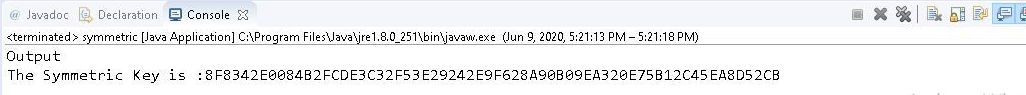Encryption and Decryption using the symmetric key: The following steps can be followed in order to perform the encryption and decryption.

• Create the Initialization vector that is required to avoid repetition during the encryption process. This is basically a random number.
• The cipher class provides two functionalities the Encryption and Decryption. It can used to specify two different modes.

Cipher cipher = Cipher.getInstance(“AES/CBC/PKCS5Padding”);
cipher.init(Cipher.ENCRYPT_MODE, secretKey, ivParameterSpec);
cipher.init(Cipher.DECRYPT_MODE, secretKey, ivParameterSpec);

• Finally doFinal() method is invoked on cipher which Encrypts or decrypts data in a single-part operation, or finishes a multiple-part operation and returns a byte array.

doFinal(byte[] input)

Below is the implementation of the symmetric encryption and decryption. Here, we are using AES(ADVANCED ENCRYPTION STANDARD) algorithm to perform the encryption.

## Java

 `// Java program to implement the``// encryption and decryption` `import` `java.security.SecureRandom;``import` `java.util.Scanner;` `import` `javax.crypto.Cipher;``import` `javax.crypto.KeyGenerator;``import` `javax.crypto.SecretKey;``import` `javax.crypto.spec``    ``.IvParameterSpec;``import` `javax.xml.bind``    ``.DatatypeConverter;` `// Creating the symmetric``// class which implements``// the symmetric``public` `class` `symmetric {` `    ``private` `static` `final` `String AES``        ``= ``"AES"``;` `    ``// We are using a Block cipher(CBC mode)``    ``private` `static` `final` `String AES_CIPHER_ALGORITHM``        ``= ``"AES/CBC/PKCS5PADDING"``;` `    ``private` `static` `Scanner message;` `    ``// Function to create a``    ``// secret key``    ``public` `static` `SecretKey createAESKey()``        ``throws` `Exception``    ``{``        ``SecureRandom securerandom``            ``= ``new` `SecureRandom();``        ``KeyGenerator keygenerator``            ``= KeyGenerator.getInstance(AES);` `        ``keygenerator.init(``256``, securerandom);``        ``SecretKey key``            ``= keygenerator.generateKey();` `        ``return` `key;``    ``}` `    ``// Function to initialize a vector``    ``// with an arbitrary value``    ``public` `static` `byte``[] createInitializationVector()``    ``{` `        ``// Used with encryption``        ``byte``[] initializationVector``            ``= ``new` `byte``[``16``];``        ``SecureRandom secureRandom``            ``= ``new` `SecureRandom();``        ``secureRandom.nextBytes(initializationVector);``        ``return` `initializationVector;``    ``}` `    ``// This function takes plaintext,``    ``// the key with an initialization``    ``// vector to convert plainText``    ``// into CipherText.``    ``public` `static` `byte``[] do_AESEncryption(``        ``String plainText,``        ``SecretKey secretKey,``        ``byte``[] initializationVector)``        ``throws` `Exception``    ``{``        ``Cipher cipher``            ``= Cipher.getInstance(``                ``AES_CIPHER_ALGORITHM);` `        ``IvParameterSpec ivParameterSpec``            ``= ``new` `IvParameterSpec(``                ``initializationVector);` `        ``cipher.init(Cipher.ENCRYPT_MODE,``                    ``secretKey,``                    ``ivParameterSpec);` `        ``return` `cipher.doFinal(``            ``plainText.getBytes());``    ``}` `    ``// This function performs the``    ``// reverse operation of the``    ``// do_AESEncryption function.``    ``// It converts ciphertext to``    ``// the plaintext using the key.``    ``public` `static` `String do_AESDecryption(``        ``byte``[] cipherText,``        ``SecretKey secretKey,``        ``byte``[] initializationVector)``        ``throws` `Exception``    ``{``        ``Cipher cipher``            ``= Cipher.getInstance(``                ``AES_CIPHER_ALGORITHM);` `        ``IvParameterSpec ivParameterSpec``            ``= ``new` `IvParameterSpec(``                ``initializationVector);` `        ``cipher.init(``            ``Cipher.DECRYPT_MODE,``            ``secretKey,``            ``ivParameterSpec);` `        ``byte``[] result``            ``= cipher.doFinal(cipherText);` `        ``return` `new` `String(result);``    ``}` `    ``// Driver code``    ``public` `static` `void` `main(String args[])``        ``throws` `Exception``    ``{``        ``SecretKey Symmetrickey``            ``= createAESKey();` `        ``System.out.println(``            ``"The Symmetric Key is :"``            ``+ DatatypeConverter.printHexBinary(``                  ``Symmetrickey.getEncoded()));` `        ``byte``[] initializationVector``            ``= createInitializationVector();` `        ``String plainText``            ``= ``"This is the message "``              ``+ ``"I want To Encrypt."``;` `        ``// Encrypting the message``        ``// using the symmetric key``        ``byte``[] cipherText``            ``= do_AESEncryption(``                ``plainText,``                ``Symmetrickey,``                ``initializationVector);` `        ``System.out.println(``            ``"The ciphertext or "``            ``+ ``"Encrypted Message is: "``            ``+ DatatypeConverter.printHexBinary(``                  ``cipherText));` `        ``// Decrypting the encrypted``        ``// message``        ``String decryptedText``            ``= do_AESDecryption(``                ``cipherText,``                ``Symmetrickey,``                ``initializationVector);` `        ``System.out.println(``            ``"Your original message is: "``            ``+ decryptedText);``    ``}``}`

Output: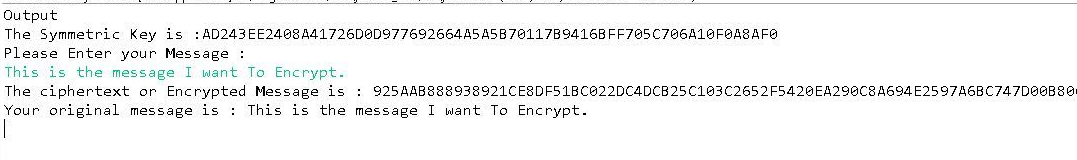My Personal Notes arrow_drop_up